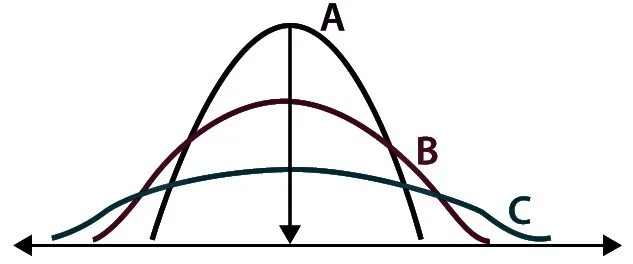# Absolute and Relative Measures

27th January 2021

The measure of dispersion indicates the scattering of data. It explains the disparity of data from one another, delivering a precise view of the distribution of data. The measure of dispersion displays and gives us an idea about the variation and central value of an individual item.Characteristics of a Good Measure of Dispersion

• It should be easy to calculate & simple to understand.
• It should be based on all the observations of the series.
• It should be rigidly defined.
• It should not be affected by extreme values.
• It should not be unduly affected by sampling fluctuations.
• It should be capable of further mathematical treatment and statistical analysis.

Relative Measure of Dispersion

• Relative measures of dispersion are obtained as ratios or percentages of the average.
• These are also known as ‘Coefficient of dispersion.’
• These are pure numbers or percentages totally independent of the units of measurements.

The relative measures of depression are used to compare the distribution of two or more data sets. This measure compares values without units. Common relative dispersion methods include:

• Co-efficient of Range
• Co-efficient of Variation
• Co-efficient of Standard Deviation
• Co-efficient of Quartile Deviation
• Co-efficient of Mean Deviation

Absolute Measure of Dispersion

An absolute measure of dispersion contains the same unit as the original data set. Absolute dispersion method expresses the variations in terms of the average of deviations of observations like standard or means deviations. It includes range, standard deviation, quartile deviation, etc.

The types of absolute measures of dispersion are:

• Range: It is simply the difference between the maximum value and the minimum value given in a data set. Example: 1, 3,5, 6, 7 => Range = 7 -1= 6
• Variance: Deduct the mean from each data in the set then squaring each of them and adding each square and finally dividing them by the total no of values in the data set is the variance. Variance (σ2)=∑(X−μ)2/N
• Standard Deviation: The square root of the variance is known as the standard deviation i.e. S.D. = √σ.
• Quartiles and Quartile Deviation: The quartiles are values that divide a list of numbers into quarters. The quartile deviation is half of the distance between the third and the first quartile.
• Mean and Mean Deviation: The average of numbers is known as the mean and the arithmetic mean of the absolute deviations of the observations from a measure of central tendency is known as the mean deviation (also called mean absolute deviation).